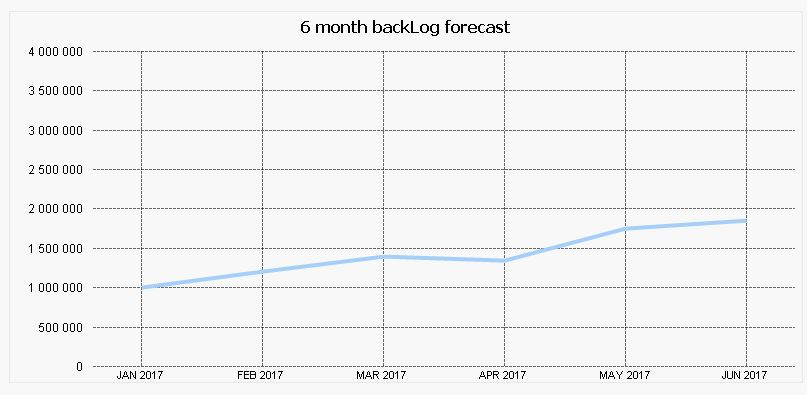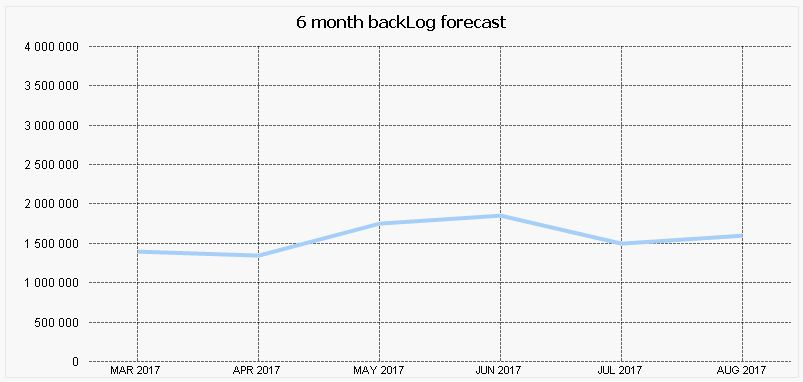# QlikView App Dev

Discussion Board for collaboration related to QlikView App Development.

Announcements
cancel
Showing results for
Did you mean:Creator II

## 6 month rolling forecast

Hi All,

Here is the issue i am facing :

i have a database showing for each month a sales forecast :The idea is to show a 6 month forecast based on the period selected

For instance, if December 2016 is selected, the outpout should be the data for the 6 following period (from January to June 2017) as seen below :If February 2017 is selected, then the output should be the data from March to August 2017 as seen below :For those who want to help, let me attached the Excel test database as well as the .qvw file

Guillaume

1 Solution

Accepted SolutionsChampion III

sum({<Année_Mois={">\$(=max(Année_Mois))<\$(=addmonths(max(Année_Mois),6))"},[\$(=concat({<\$Table={'Calendrier'},\$Field-={'Année_Mois'}>} \$Field,'],['))]>} [Forecast Sales Zone 1])

The concat() part of the expression is perhaps overkill, but it lets you add or remove Calendrier fields without having to modify the expression.

However, you might consider using an AsOf table connecting each month to the 6 future months. Much simpler expression. But there can then be confusion about which field is being selected, and if the wrong one is selected, strange results can happen.

AsOf_Mois, Année_Mois
Feb 2017, Mar 2017
Feb 2017, Apr 2017
...
Feb 2017, Aug 2017

Dimension  = AsOf_Mois
Expression = sum([Forecast Sales Zone 1])

The As-Of Table

2 RepliesChampion III

sum({<Année_Mois={">\$(=max(Année_Mois))<\$(=addmonths(max(Année_Mois),6))"},[\$(=concat({<\$Table={'Calendrier'},\$Field-={'Année_Mois'}>} \$Field,'],['))]>} [Forecast Sales Zone 1])

The concat() part of the expression is perhaps overkill, but it lets you add or remove Calendrier fields without having to modify the expression.

However, you might consider using an AsOf table connecting each month to the 6 future months. Much simpler expression. But there can then be confusion about which field is being selected, and if the wrong one is selected, strange results can happen.

AsOf_Mois, Année_Mois
Feb 2017, Mar 2017
Feb 2017, Apr 2017
...
Feb 2017, Aug 2017

Dimension  = AsOf_Mois
Expression = sum([Forecast Sales Zone 1])

The As-Of TableCreator II
Author

Thanks a lot John !